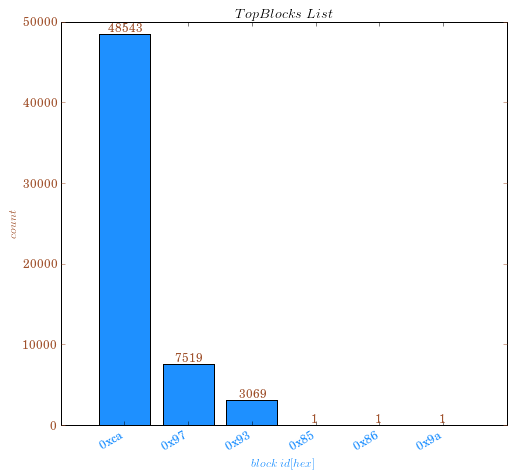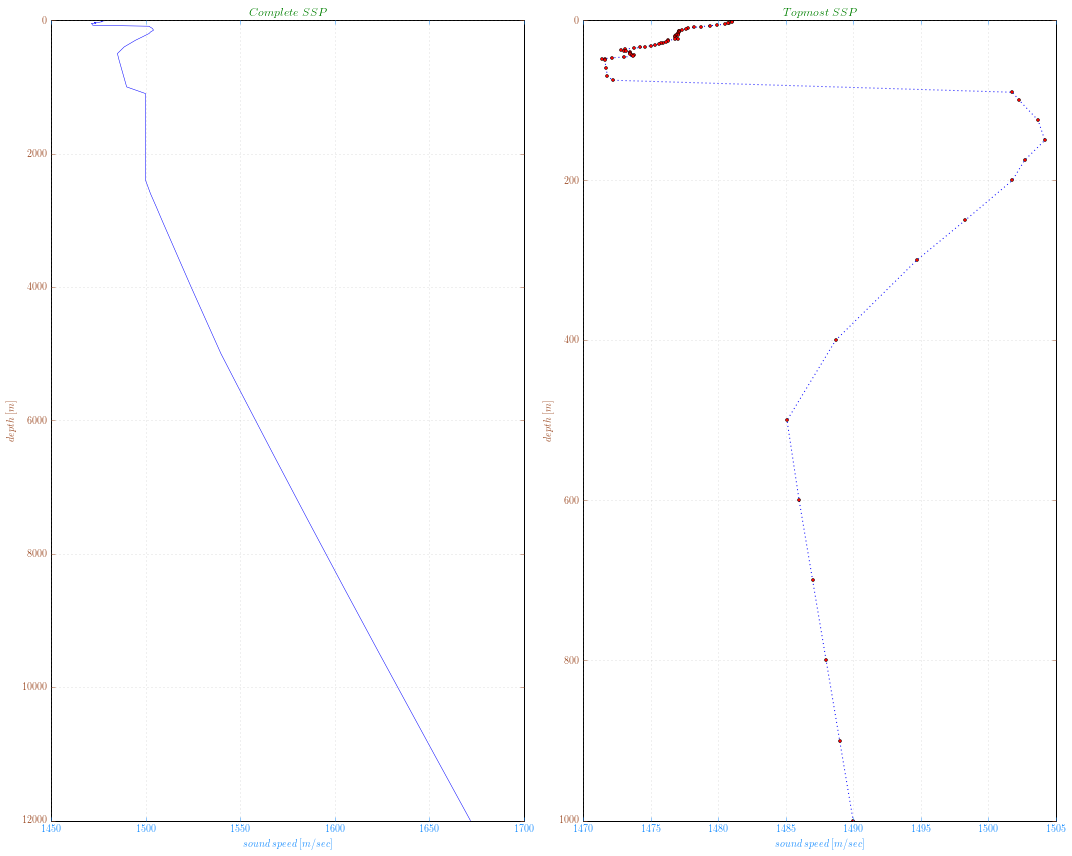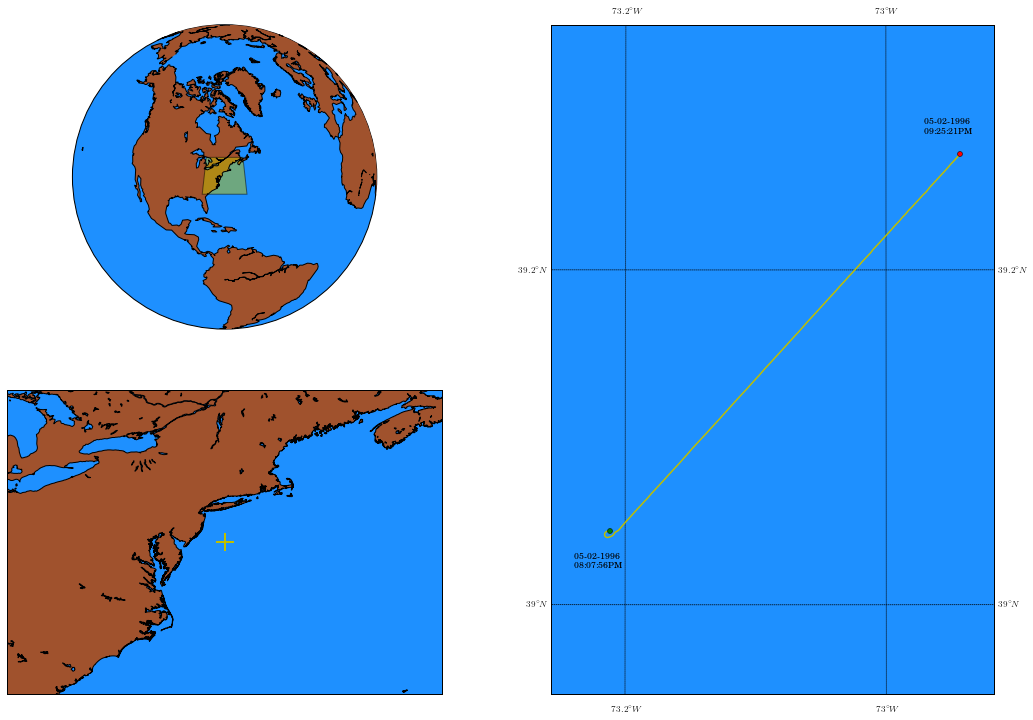HydrOffice news

## Digging into the past: Huddl for legacy data formats

Feb. 10, 2015, 2:40 p.m., author: gmasetti

## Introduction¶

Data acquired during a hydrographic survey may be stored in a number of different formats. Essentially, every manufacturer has developed its own format specifications, and development during the lifetime of existing or new acquisition systems usually requires a sequence of file format releases. Trying to keep abreast of all of the different formats, their change-points, and idiosyncrasies, can be a time-consuming and problematic endeavor for anyone who has to read multiple different data formats, or deal with archival data.

One of the biggest (and negative) consequences of the current situation is that each data handling application has its own data parsers (coded mostly from scratch) for every supported data format, and these parsers must keep up to date. This is a significant resource sink that could be reduced, and entails the danger of allowing variant data content interpretations in different software packages. Survey data access becomes still more complicated if the source files are stored in legacy data formats, where negotiation of multiple versions of even one format may be required if historical trend analysis is the primary goal. A useful solution to survey data access would be to offer access to mixed-format historical data, providing a mechanism to describe data collected and archived in sometimes ‘exotic’ data formats (e.g., developed by now defunct manufacturers). Solving this issue implies the definition of a reliable way to access the data collected today by our descendants, with obvious advantages in the adoption of these methods by hydrographic data archiving centers.

The key point of the Huddl approach is to describe the existing formats as they are, rather than define another chimeric format able to encapsulate the amount of information present in all the existing data formats (with all the related semantic issues in case a conversion is attempted). At the same time, Huddl provides an inexpensive but robust way to deal with many legacy data formats. When required to access old datasets in an arbitrary binary format, an ad hoc Huddl Format Description (HFD) may be created that can deal with the particular vagueness of some legacy formats or some rare variant implementations. As a working example on the use of Huddl in support of legacy data formats, this post uses Huddl technology to access data collected in 1996 with a Kongsberg EM 950 MBES.

The EM 950, characterized by a curved transducer, was one of the first high frequency Kongsberg multibeam echo sounder. A few years ago the manufacturer stopped its general maintenance.

## Python script¶

The Huddl-based Python module for the Kongsberg EM 950 data format was created using the same approach described in these previous two posts:

Once created, the module is imported to be used in a Python script in order to access the information present in a legacy data file.

The script also imports several commonly used modules such as `matplotlib` and `mpl_toolkits.basemap` (for plotting utilities), the powerful `numpy`, and some standard Python libraries such as `datetime` and `operator`.

In :
```import kongsberg_em1000 as em1k
import os.path
import datetime
import pytz
import operator
import matplotlib
from matplotlib import pyplot as plt
from matplotlib import rcParams
from matplotlib import ticker
from mpl_toolkits.basemap import Basemap
from matplotlib.path import Path
import matplotlib.patches as patches
import numpy as np```

Some simple helper classes and methods are used to store and manage the data content:

In :
```class Em1kSsp:
depths = np.zeros(0, dtype=np.float)
values = np.zeros(0, dtype=np.float)

class Em1kPos:
times = np.zeros(0, dtype='datetime64[us]')
lats = np.zeros(0, dtype=np.float)
longs = np.zeros(0, dtype=np.float)

class Em1kData:
""" container for Kongsberg EM1000 data """
ssp_list = list()
nav = Em1kPos()

def make_time(date, time):
""" helper function to retrieve date and time """
# date
datetime_str = str()
count = 0
while True:
break
count += 1
count = 0

#time
while True:
break
count += 1

dt = datetime.datetime.strptime(datetime_str, "%d%m%y%H%M%S%f")
return dt.replace(tzinfo=pytz.UTC)

def make_fix(lng, ltd):
""" helper function to retrieve position as longitude and latitude in decimal degrees """
lat_dd = int(chr(em1k.u8_array_getitem(ltd, 0)) + chr(em1k.u8_array_getitem(ltd, 1)))
long_dd = int(chr(em1k.u8_array_getitem(lng, 0)) + chr(em1k.u8_array_getitem(lng, 1)) +
chr(em1k.u8_array_getitem(lng, 2)))

lat_mm = float(chr(em1k.u8_array_getitem(ltd, 2)) + chr(em1k.u8_array_getitem(ltd, 3)) + '.' +
chr(em1k.u8_array_getitem(ltd, 5)) + chr(em1k.u8_array_getitem(ltd, 6)) +
chr(em1k.u8_array_getitem(ltd, 7)) + chr(em1k.u8_array_getitem(ltd, 8)))

long_mm = float(chr(em1k.u8_array_getitem(lng, 3)) + chr(em1k.u8_array_getitem(lng, 4)) + '.' +
chr(em1k.u8_array_getitem(lng, 6)) + chr(em1k.u8_array_getitem(lng, 7)) +
chr(em1k.u8_array_getitem(lng, 8)) + chr(em1k.u8_array_getitem(lng, 9)))

lat = lat_dd + (lat_mm / 60.0)
if chr(em1k.u8_array_getitem(ltd, 9)) == 'S':
lat = -lat
long = long_dd + (long_mm / 60.0)
if chr(em1k.u8_array_getitem(lng, 10)) == 'W':
long = -long

return long, lat```

### Content Exploration¶

Following an approach similar to the one explained in this post, during file traversal, we collect information about the avaialable `TopBlock`s. These functions are used to list the collected information:

In :
```def print_info(datagram_nr, datagram_dict):
""" Provide some info about the data file """
print("\n> data file content:")
print("  . total blocks:\t", datagram_nr)
print("  . blocks list:")
print("  [type] --> [counter]")
for d in reversed(sorted(datagram_dict, key=datagram_dict.get)):
print("   #%s --> %s" % (hex(d), datagram_dict[d]))
print("")

def plot_info(datagram_dict):
""" Plot the blocks list as bar chart ordered from the most common """
keys, values = zip(*sorted(datagram_dict.items(), key=operator.itemgetter(1), reverse=True))
fig, ax0 = plt.subplots(ncols=1, nrows=1, figsize=(8, 8), dpi=100)
matplotlib.rcParams.update({'font.size': 12, 'text.usetex': True, 'font.family': 'serif'})
bar = ax0.bar(range(len(values)), values, align='center', color='#1e90ff')
# attach some text labels
for rect in bar:
height = rect.get_height()
ax0.text(rect.get_x()+rect.get_width()/2., height + 100, '%d'%int(height), ha='center', va='bottom', color='#a0522d')
ax0.set_title('\$TopBlocks\$  \$List\$')
ax0.tick_params(axis='y', colors='#a0522d')
ax0.set_ylabel('\$count\$', color='#a0522d')
ax0.set_xlabel('\$block\$ \$id[hex]\$', color='#1e90ff')
ax0.set_xticks(range(len(keys)))
ax0.set_xticklabels([hex(lab) for lab in keys], color='#1e90ff')
fig.autofmt_xdate()
plt.show()
fig.savefig('em1k_datagrams', dpi=600)```

### Sound Speed Profiles¶

Large amount of legacy data have a big potential that is too often hidden by the difficulties in physically accessing their content. A basic example is sound speed profiles (SSPs) stored in some legacy data formats. The following functions are used to read, print, and plot the SSPs stored in the example data file:

In :
```def read_svp(data_header, data_content, em1k_data):
""" read and store a Kongsberg svp datagram """
nr_entries = data_content.svp.n_values
svp = Em1kSsp()
svp.depths = np.zeros(nr_entries, dtype=np.float)
svp.values = np.zeros(nr_entries, dtype=np.float)

for i in range(nr_entries):
loc_svp = em1k.em91svpentry_array_getitem(data_content.svp.svp, i)
svp.depths[i] = loc_svp.depth
svp.values[i] = loc_svp.speed / 10.0

em1k_data.ssp_list.append(svp)

def print_svp(em1k_data):
""" print the read Kongsberg SSP data """
print("> Sound speed profiles: ", len(em1k_data.ssp_list))
count = 0
for profile in em1k_data.ssp_list:
print("  # profile ", count)
for i in range(len(profile.depths)):
if (i < 5) or (i > len(profile.depths)-5):
print("  .\t%04d" % i, "\t", profile.depths[i], "\t%4.2f" % profile.values[i])
if i == 5:
print("  .\t...")
count += 1

def plot_svp(em1k_data):
""" plot and save the read Kongsberg SSP data """

matplotlib.rcParams.update({'font.size': 10, 'text.usetex': True, 'font.family': 'serif'})

count = 0
for profile in em1k_data.ssp_list:

fig, (ax0, ax1) = plt.subplots(ncols=2, nrows=1, figsize=(15, 12), dpi=100)

plot_ssp = ax0.plot(profile.values, profile.depths, linewidth=0.5)
ax0.grid(color='gray', alpha=0.5, linewidth=0.5)
ax0.set_title('\$Complete\$ \$SSP\$')
ax0.title.set_color('green')
ax0.set_ylabel('\$depth\$ \$[m]\$', color='#a0522d')
ax0.tick_params(axis='y', colors='#a0522d')
ax0.set_xlabel('\$sound\$ \$speed\$ \$[m/sec]\$', color='#1e90ff')
ax0.tick_params(axis='x', colors='#1e90ff')
ax0.invert_yaxis()

plot_ssp = ax1.plot(profile.values[0:68], profile.depths[0:68], ':b')
plot_ssp = ax1.plot(profile.values[0:68], profile.depths[0:68], 'ro', markersize=3)
ax1.grid(color='gray', alpha=0.5, linewidth=0.5)
ax1.set_title('\$Topmost\$ \$SSP\$')
ax1.title.set_color('green')
ax1.set_ylabel('\$depth\$ \$[m]\$', color='#a0522d')
ax1.tick_params(axis='y', colors='#a0522d')
ax1.set_xlabel('\$sound\$ \$speed\$ \$[m/sec]\$', color='#1e90ff')
ax1.tick_params(axis='x', colors='#1e90ff')
ax1.invert_yaxis()

plt.tight_layout()
plt.show()
fig.savefig('em1k_svp', dpi=600)

count += 1```

As a more general application, the position fixes present in the survey line are extracted, and then they are plotted on a geographic map:

In :
```def read_nav(data_header, data_content, em1k_data):
""" read and store a Kongsberg nav datagram """

# retrieve date and time
dt = make_time(data_content.position.date, data_content.position.time)

# retrieve position
long, lat = make_fix(data_content.position.longitude, data_content.position.latitude)

# store retrieved information
em1k_data.nav.times = np.append(em1k_data.nav.times, dt)
em1k_data.nav.longs = np.append(em1k_data.nav.longs, long)
em1k_data.nav.lats = np.append(em1k_data.nav.lats, lat)

def print_nav(em1k_data):
for i in range(len(em1k_data.nav.times)):
if (i < 5) or (i > len(em1k_data.nav.times)-5):
print("#%04d" % i, "\t", em1k_data.nav.times[i],
"\t%3.7f" % em1k_data.nav.longs[i],
"\t%2.7f" % em1k_data.nav.lats[i])
if i == 5:
print("# ...")

def plot_nav(em1k_data):

# bounding box
ll_mean = np.mean(em1k_data.nav.longs)
lt_mean = np.mean(em1k_data.nav.lats)

# creates axes
fig = plt.figure(figsize=(18, 12), dpi=100)
ax1 = plt.subplot2grid((2, 2), (0, 0))
ax2 = plt.subplot2grid((2, 2), (1, 0))
ax3 = plt.subplot2grid((2, 2), (0, 1), rowspan=2)

# make background maps
map1 = Basemap(projection='ortho', lon_0=ll_mean, lat_0=lt_mean, ax=ax1)
map1.drawmapboundary(fill_color='#1e90ff')
map1.fillcontinents(color='#a0522d', lake_color='#1e90ff')
map1.drawcoastlines()
map2 = Basemap(projection='cyl', resolution='h', ax=ax2,
llcrnrlon=ll_mean-10.0, llcrnrlat=lt_mean-7., urcrnrlon=ll_mean+10.0, urcrnrlat=lt_mean+7.)
map2.drawmapboundary(fill_color='#1e90ff')
map2.fillcontinents(color='#a0522d', lake_color='#1e90ff')
map2.drawcoastlines()
map3 = Basemap(projection='tmerc', lon_0=ll_mean, lat_0=lt_mean, ax=ax3,
llcrnrlon=ll_mean-0.17, llcrnrlat=lt_mean-0.2, urcrnrlon=ll_mean+0.17, urcrnrlat=lt_mean+0.2)
map3.drawmapboundary(fill_color='#1e90ff')
map3.fillcontinents(color='#a0522d', lake_color='#1e90ff')
map3.drawcoastlines()
map3.drawparallels(np.arange(0., 90, 0.2), labels=[1, 1, 0, 0], fontsize=9, alpha=0.9)
map3.drawmeridians(np.arange(180., 360., 0.2), labels=[0, 0, 1, 1], fontsize=9, alpha=0.9)

# drawing zoom area
lbx1, lby1 = map1(*map2(map2.xmin, map2.ymin, inverse=True))
ltx1, lty1 = map1(*map2(map2.xmin, map2.ymax, inverse=True))
rtx1, rty1 = map1(*map2(map2.xmax, map2.ymax, inverse=True))
rbx1, rby1 = map1(*map2(map2.xmax, map2.ymin, inverse=True))
verts1 = [(lbx1, lby1),       # left, bottom
(ltx1, lty1),       # left, top
(rtx1, rty1),       # right, top
(rbx1, rby1),       # right, bottom
(lbx1, lby1), ]     # ignored
codes2 = [Path.MOVETO, Path.LINETO, Path.LINETO, Path.LINETO, Path.CLOSEPOLY, ]
path = Path(verts1, codes2)
patch = patches.PathPatch(path, facecolor='y', lw=1, alpha=0.5)

# drawing survey line as a single point
x, y = map2(ll_mean, lt_mean)
map2.plot(x, y, 'y+', markersize=18, mew=2.0)

#drawing survey line as connected fixes
x, y = map3(em1k_data.nav.longs, em1k_data.nav.lats)
map3.plot(x, y, 'y-', lw=1.5)
map3.plot(x, y, 'go', markersize=5)
plt.text(x-2400, y-2400, '%s' % em1k_data.nav.times.strftime("%m-%d-%Y\n%I:%M:%S%p"), fontsize=9)
map3.plot(x[-1], y[-1], 'ro', markersize=5)
plt.text(x[-1]-2400, y[-1]+1400, '%s' % em1k_data.nav.times[-1].strftime("%m-%d-%Y\n%I:%M:%S%p"), fontsize=9)

plt.show()
fig.savefig('em1k_nav', dpi=600)```

### Data access and visualization¶

The reading mechanism to access the data file content is quite simple, and it has already been presented here. We first open the raw file, then we check if the opening has been successful:

In :
```# Open the data file
testfile_path = "C:/.../surveyline.all"
if not os.path.isfile(testfile_path):
raise IOError("the file %s does not exist" % testfile_path)
file_size = os.path.getsize(testfile_path)
ip = em1k.fopen(testfile_path, "rb")
if ip is None:
raise IOError("data file not successfully open")
print("> data file successfully open")
position = em1k.ftell(ip)
print("> initial position: ", position)```

```> data file successfully open
> initial position:  0```

Some variables are declared here that will be populated in the next code snippets:

In :
```# counters, block dictionary and other information
datagram_dict = dict()
em1k_data = Em1kData()
datagram_nr = 0

We now use a loop that goes through all the content datagrams looking for datagram types and counting them using the previously declared dictionary. In addition, if the `TopBlock` read stores navigation information or SSP, the previosuly introduced functions are called:

In :
```# Loop to read all the datagrams
abort = False
resync_event = 0
em1k.fseek(ip, 0, 0) # to go back at the beginning of data file
print("> looping through the whole data file: ", n_read)
while not abort:

if rc == em1k.HUDDLER_TOPBLOCK_OK:
if datagram_nr < 5: # to print only the first blocks
datagram_nr += 1

elif rc == em1k.HUDDLER_TOPBLOCK_UNKNOWN:
print("> #", datagram_nr, " -> UNKNOWN datagram ", hex(data.header.type), " at offset ", position)
datagram_nr += 1
em1k.fseek(ip, 1, 1)                        # Move on by one --- no length

print("> #", datagram_nr, " -> no header at offset ", position, " -> resynch #", resync_event)
resync_event += 1

print("> #", datagram_nr, " -> fail reading datagram at offset ", position, " -> resynch #", resync_event)
resync_event += 1

elif rc == em1k.HUDDLER_INVALID_TAIL:
print("> #", datagram_nr, " -> invalid tail at offset ", position, " -> resynch #", resync_event)
resync_event += 1

elif rc == em1k.HUDDLER_EOF_WHILE:
print("  . . .\n"
"  #", datagram_nr, "->\tend of file at offset ", position)
abort = True

print("> #", datagram_nr, " -> unexpected read error at offset ", position, " -> aborting")
abort = True

else:
print("> unknown read return: ", rc)
abort = True

position = em1k.ftell(ip)

# Closing the data source
print("> last position read in file: ", position, " [file size: ", file_size, "]")
if position == file_size:
print("> the whole data file has been traversed")
else:
print("> WARNING: the whole data file has NOT been traversed !!")
ret = em1k.fclose(ip)
if ret == 0:
print("> data file closed")```

```> looping through the whole data file:  0
# 0 	->	bytes:  426 	type:  133 [ 0x85 ]
# 1 	->	bytes:  421 	type:  154 [ 0x9a ]
# 2 	->	bytes:  556 	type:  202 [ 0xca ]
# 3 	->	bytes:  556 	type:  202 [ 0xca ]
# 4 	->	bytes:  556 	type:  202 [ 0xca ]
. . .
# 59134 ->	end of file at offset  32523479
> last position read in file:  32523479  [file size:  32523479 ]
> the whole data file has been traversed
> data file closed```

All the `TopBlock`s in the data file have been visited (we limited the visualization to just the first 5 datagrams). As a double check, we compare the file size with the number of bytes read, and they match.

The helper functions `print_info` and `plot_info` are then called so that the user can inspect the file content:

In :
```%matplotlib inline
print_info(datagram_nr, datagram_dict)
plot_info(datagram_dict)```

```> data file content:
. total blocks:	 59134
. blocks list:
[type] --> [counter]
#0xca --> 48543
#0x97 --> 7519
#0x93 --> 3069
#0x9a --> 1
#0x86 --> 1
#0x85 --> 1```From the bar chart, we discover that the file contains 3069 position fixes (type `0x93`) and 1 SSP (type `0x9a`). We decide to inspect the profile to have a first visual evaluation of the quality (e.g., absence of spikes):

In :
```print_svp(em1k_data)
plot_svp(em1k_data)```

```> Sound speed profiles:  1
# profile  0
.	0000 	 1.0 	1481.00
.	0001 	 2.0 	1481.00
.	0002 	 3.0 	1480.80
.	0003 	 4.0 	1480.70
.	0004 	 5.0 	1480.50
.	...
.	0096 	 10000.0 	1633.40
.	0097 	 10500.0 	1643.00
.	0098 	 11000.0 	1652.60
.	0099 	 12000.0 	1671.90```The left panel in the plot shows the full profile, the profile contains 100 samples and their density is much higher in the first few meters (As required by the acquisition software, the profile has been artificially extended to 12000 meters). However, the part that is usually of more scientific interest is usually the topmost, so we displayed the first 1000 meters on the right panel.

Finally, we plot the navigation of the legacy survey line.

In :
```print_nav(em1k_data)
plot_nav(em1k_data)```

```> Navigation fixes:  3069
#0000 	 1996-05-02 20:07:56.780000+00:00 	-73.2117317 	39.0439083
#0001 	 1996-05-02 20:07:58.770000+00:00 	-73.2119217 	39.0438900
#0002 	 1996-05-02 20:07:59.780000+00:00 	-73.2120167 	39.0438833
#0003 	 1996-05-02 20:08:01.780000+00:00 	-73.2122017 	39.0438783
#0004 	 1996-05-02 20:08:04.780000+00:00 	-73.2124717 	39.0438850
# ...
#3065 	 1996-05-02 21:25:18.750000+00:00 	-72.9430717 	39.2690950
#3066 	 1996-05-02 21:25:19.750000+00:00 	-72.9430100 	39.2691517
#3067 	 1996-05-02 21:25:20.750000+00:00 	-72.9429500 	39.2692083
#3068 	 1996-05-02 21:25:21.750000+00:00 	-72.9428883 	39.2692633```In :
`print("Done")`

`Done`

tags: notebook; huddl; legacy